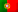Home > Technology > Computer Science > Programming > C++ > Function templates in C++

# Function templates in C++

In a previous article, I talked about a class template in C++. Now, I’m going to talk about function templates. A function template is very similar to a class template, allowing the definition of generic types in its return value and/or parameters. And its code will also be generated only when the compiler founds the usage of these functions.

To male a parallelism with the class template from the previous article, we are going to see two functions that will receive two generic type values and, one will return which is the greater value (max) and the other, which is the lesser value (min).

```template <typename T>
T max(T &val1, T &val2) {
return (val1 > val2 ? val1 : val2);
}
```

In the first line, with the declaration “template <typename T>”, we are telling the compiler we are going to use a template with a generic type “T“. This “T” is used to specify the return value data type and for the parameters (val1 and val2), as we can see in line 2. And notice too the usage of the character “&” in the parameters, because in a function template, we should tell the compiler if we want the address of a variable or a pointer in the parameters. If you don’t do this, when we call this function, passing variables as parameters, the compiler will throw an error saying the call to the function is ambiguous.

Let’s now see an example of a call to this function, assuming we have two integer variables, i1 and i2:

```    cout << "The greater integer is: " << max(i1, i2) << endl;
```

Let’s see the code for the function “min”:

```template <typename T>
T min(T &val1, T &val2) {
return (val1 < val2 ? val1 : val2);
}
```

Similar to function “max”, we are telling the compiler again we have a template with a generic type “T”. Let’s see how to call this function with the integers i1 and i2:

```    cout << "The lesser integer is: " << min(i1, i2) << endl;
```

The following complete program will use three different data types for the functions “max” and “min”:

```#include <iostream>
#include <stdlib.h>
#include <string>

using namespace std;

template <typename T>
T max(T &val1, T &val2) {
return (val1 > val2 ? val1 : val2);
}

template <typename T>
T min(T &val1, T &val2) {
return (val1 < val2 ? val1 : val2);
}

int main() {
int i1, i2;
float f1, f2;
string s1, s2;
cout << "Insert 2 integers:" << endl;
cin >> i1;
cin >> i2;
cout << "The greater integer is: " << max(i1, i2) << endl;
cout << "The lesser integer is: " << min(i1, i2) << endl;
cout << "Insert 2 floats:" << endl;
cin >> f1;
cin >> f2;
cout << "The greater float is: " << max(f1, f2) << endl;
cout << "the lesser float is: " << min(f1, f2) << endl;
cout << "Insert 2 strings:" << endl;
cin >> s1;
cin >> s2;
cout << "The greater string is: " << max(s1, s2) << endl;
cout << "The lesser string is: " << min(s1, s2) << endl;
}
```

Let’s see an example of the execution of this code:

Insert 2 integers:
120
90
The greater integer is: 120
The lesser integer is: 90
Insert 2 floats:
14.5
16.7
The greater float is: 16.7
the lesser float is: 14.5
Insert 2 strings:
def
abc
The greater string is: def
The lesser string is: abc

Follow us on the social networks and stay tuned with news from our multi-thematic blog – Out4Mind!